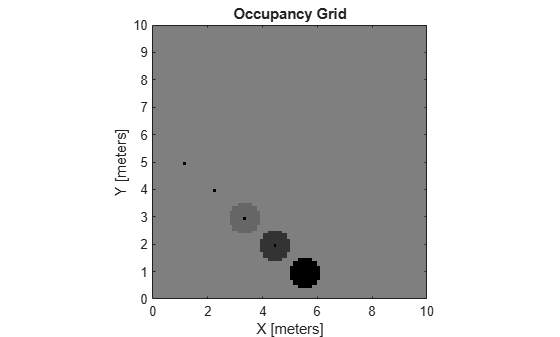inflate

Inflate each occupied grid location

Description

example

inflate(map,radius) inflates each occupied position of the specified map by the radius, specified in meters. Occupied location values are based on the map.OccupiedThreshold property. radius is rounded up to the nearest equivalent cell based on the resolution of the map. Values are modified using grayscale inflation to inflate higher probability values across the grid. This inflation increases the size of the occupied locations in the map.

inflate(map,gridradius,'grid') inflates each occupied position by the gridradius, specified in number of cells.

Examples

collapse all

Create an empty map of 10-by-10 meters in size.

map = occupancyMap(10,10,10);

Update the occupancy of specific world locations with new probability values and display the map.

x = [1.2; 2.3; 3.4; 4.5; 5.6];
y = [5.0; 4.0; 3.0; 2.0; 1.0];

pvalues = [0.2; 0.4; 0.6; 0.8; 1];

updateOccupancy(map,[x y],pvalues)
figure
show(map)Inflate the occupied areas by a radius of 0.5 m. The larger occupancy values overwrite the smaller values.

inflate(map,0.5)
figure
show(map)Get the grid locations from the world locations.

ij = world2grid(map,[x y]);

Set occupancy values for the grid locations.

setOccupancy(map,ij,ones(5,1),'grid')
figure
show(map)Input Arguments

collapse all

Map representation, specified as a occupancyMap object. This object represents the environment of the vehicle. The object contains a matrix grid with values representing the probability of the occupancy of that cell. Values close to 1 represent a high probability that the cell contains an obstacle. Values close to 0 represent a high probability that the cell is not occupied and obstacle free.

Dimension that defines by how much to inflate occupied locations, specified as a scalar in meters. radius is rounded up to the nearest equivalent cell value.

Data Types: double

Number of cells by which to inflate the occupied locations, specified as a positive integer scalar.

Data Types: double

collapse all

Grayscale Inflation

In grayscale inflation, the strel (Image Processing Toolbox) function creates a circular structuring element using the inflation radius. The grayscale inflation of A(x, y) by B(x, y) is defined as:

(AB)(x, y) = max {A(xx′, y’y′) +B(x', y') | (x′, y′) ∊ DB}.

DB is the domain of the probability values in the structuring element B. A(x,y) is assumed to be +∞ outside the domain of the grid.

Grayscale inflation acts as a local maximum operator and finds the highest probability values for nearby cells. The inflate method uses this definition to inflate the higher probability values throughout the grid. This inflation increases the size of any occupied locations and creates a buffer zone for vehicles to use as they navigate.